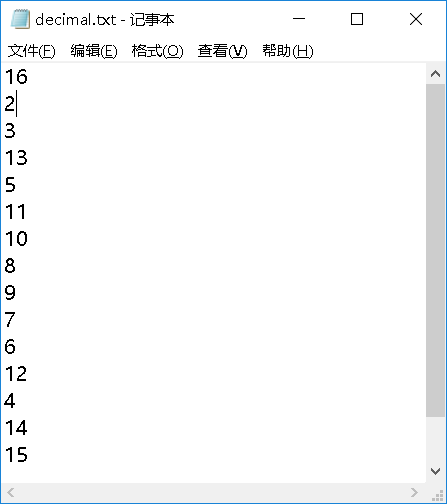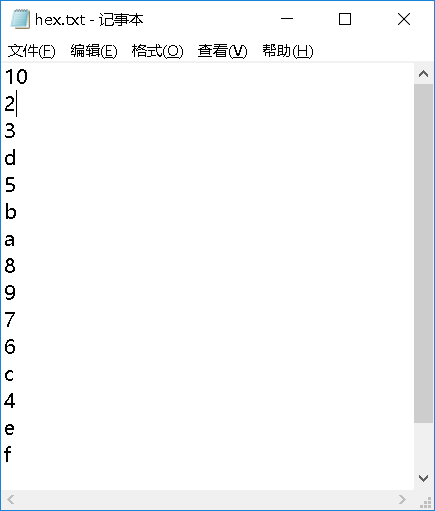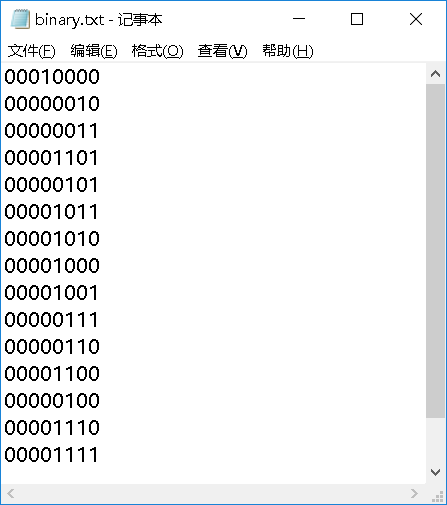• 我最近在做嵌入式图像处理，我的任务是：首先要把一幅图像读入matlab，获取每个像素点的灰度，然后分别在TXT文件中以二进制、十进制和十六进制的数值显示出来。 在matlab中使用imread函数读入一幅图像，得到由...
我最近在做嵌入式图像处理，我的任务是：首先要把一幅图像读入matlab，获取每个像素点的灰度值，然后分别在TXT文件中以二进制、十进制和十六进制的数值显示出来。

在matlab中使用imread函数读入一幅图像，得到由图像灰度构成的uint8类型的数值矩阵，然后用fopen、fclose进行文件的读写操作，用printf函数将数值打印到TXT文档中，话不多说，上代码。

由于图像数据太大，所以先写一个测试程序，这是以十进制形式输出

%% 测试程序1
close all;clear;clc;
mat=uint8(magic(4))
fid=fopen('F:\TXT测试文件\decimal.txt','wt');  %在1-decimal.txt中写入十进制数据
COUNT=fprintf(fid,'%d\n',mat');
fclose(fid);

因为matlab中图像数据读取时是按列读取和存储，而做图像处理时要按行处理，所以这里我们输出是需要将矩阵mat转置。

下面是十六进制形式输出

%% 测试程序2
close all;clear;clc;
mat=uint8(magic(4))
format hex
fid=fopen('F:\TXT测试文件\hex.txt','wt');  %在1-decimal.txt中写入十六进制数据
COUNT=fprintf(fid,'%x\n',mat');
fclose(fid);

运行结果如下但是，同样的方法输出二进制数据时却出现了问题。我在这里卡了很久，不怕大家笑话，我在这块在CSDN上找帖子学习，前后花了两周左右，总算初步学懂了几个数据I/O的函数，比如fopen、fprintf、dlmwrite，围绕这个问题还初步了解有cell、fwrite、csvwrite、xslwrite、magic、save等等。有时间再好好总结一下这次的经验，先把作业完成再说。

我想将这些数据转换成只有01的八位二进制代码，并在TXT文本中显示它们，也像上面一样一个数据一个换行。

最常用的办法是用matlab中的dec2bin函数，查看它的帮助文件。

>> help dec2bin
dec2bin Convert decimal integer to its binary representation
dec2bin(D) returns the binary representation of D as a character vector.
D must be a non-negative integer. If D is greater than flintmax,
dec2bin might not return an exact representation of D.

dec2bin(D,N) produces a binary representation with at least
N bits.

Example
dec2bin(23) returns '10111'

dec2bin(D)将矩阵D转换成二进制字符串显示,dec2bin(D,N)中N是转换后的位数。

%% 测试程序3
close all;clear;clc;
mat=uint8(magic(4))
mat1=dec2bin(mat',8)
dlmwrite('F:\TXT测试文件\binary.txt',mat1,'delimiter','','newline','pc');测试成功后用真实的图像数据试一试

clc;clear;close all;
I=imread('50.jpg');                 %读入八位十进制灰度值0-255
INFO=imfinfo('50.jpg');
R=rgb2gray(I);
%figure,imshow(I),figure,imshow(R);

%% 化成1-0黑白图像
m=graythresh(R);
BW1=im2bw(R,m);
figure,
subplot(131),imshow(BW1),title('灰度图像分为0和1');
n=graythresh(I);
BW2=im2bw(I,n);
subplot(132),imshow(BW2),title('原图分为0和1');
subplot(133),imshow(I),title('原图')

%% 以十进制字符串显示
fid=fopen('F:\360MoveData\Users\asus\Desktop\嵌入式实验\图像处理\1-decimal.txt','wt');   %在1-binary.txt中写入十进制数据
COUNT=fprintf(fid,'%d\n',R','int');%十进制数据存入TXT
sta=fclose(fid);

%% 以二进制字符串显示
B=dec2bin(R');
dlmwrite('F:\360MoveData\Users\asus\Desktop\嵌入式实验\图像处理\1-binary.txt',B,'delimiter','','newline','pc');

%% 以十六进制字符串显示
format hex         %只影响数据输出格式，不影响计算和存储
fid=fopen('F:\360MoveData\Users\asus\Desktop\嵌入式实验\图像处理\1-hex.txt','wt');      %在1-hex.txt中写入十六进制数据
COUNT2=fprintf(fid,'%x\n',R');
fclose(fid);

二进制字符显示那段代码因为数据量比较大的缘故，运行时间较长，具体时间因电脑性能而异。耐心等待一会，生成三个TXT文件，大小分别是1-binary.txt（12.5MB）、1-decimal.txt（6.00MB）、1-hex.txt（4.94MB）。打开后检查，符合要求，成功。

贴一下sprintf、fprintf的帮助

sprintf Write formatted data to string or character vector
STR = sprintf(FORMAT, A, ...) applies FORMAT to all elements of
array A and any additional array arguments in column order, and returns
the results as STR. FORMAT can be a character vector or a string
scalar. The data type of STR is the same as the data type of FORMAT.

[STR, ERRMSG] = sprintf(FORMAT, A, ...) returns an error message when
the operation is unsuccessful.  Otherwise, ERRMSG is empty.

sprintf is the same as FPRINTF except that it returns the data in a
MATLAB variable rather than writing to a file.

FORMAT describes the format of the output fields, and can include
combinations of the following:

* Conversion specifications, which include a % character, a
conversion character (such as d, i, o, u, x, f, e, g, c, or s),
and optional flags, width, and precision fields.  For more
details, type "doc sprintf" at the command prompt.

* Literal text to print.

* Escape characters, including:
\b     Backspace            ''   Single quotation mark
\f     Form feed            %%   Percent character
\n     New line             \\   Backslash
\r     Carriage return      \xN  Hexadecimal number N
\t     Horizontal tab       \N   Octal number N%
where \n is a line termination character on all platforms.

Notes:

If you apply an integer or text conversion to a numeric value that
contains a decimal, MATLAB overrides the specified conversion, and
uses %e to express the value in exponential notation.

Numeric conversions print only the real component of complex numbers.

Examples
sprintf('%0.5g',(1+sqrt(5))/2)       % 1.618
sprintf('%0.5g',1/eps)               % 4.5036e+15
sprintf('%15.5f',1/eps)              % 4503599627370496.00000
sprintf('%d',round(pi))              % 3
sprintf('%s','hello')                % hello
sprintf('The array is %dx%d.',2,3)   % The array is 2x3.

See also fprintf, sscanf, num2str, int2str, char, string, compose.

sprintf 的参考页
名为 sprintf 的其他函数


fprintf Write formatted data to text file.
fprintf(FID, FORMAT, A, ...) applies the FORMAT to all elements of
array A and any additional array arguments in column order, and writes
the data to a text file.  FID is an integer file identifier.  Obtain
FID from FOPEN, or set it to 1 (for standard output, the screen) or 2
(standard error). fprintf uses the encoding scheme specified in the
call to FOPEN.

fprintf(FORMAT, A, ...) formats data and displays the results on the
screen.

COUNT = fprintf(...) returns the number of bytes that fprintf writes.

FORMAT is a string that describes the format of the output fields, and
can include combinations of the following:

* Conversion specifications, which include a % character, a
conversion character (such as d, i, o, u, x, f, e, g, c, or s),
and optional flags, width, and precision fields.  For more
details, type "doc fprintf" at the command prompt.

* Literal text to print.

* Escape characters, including:
\b     Backspace            ''   Single quotation mark
\f     Form feed            %%   Percent character
\n     New line             \\   Backslash
\r     Carriage return      \xN  Hexadecimal number N
\t     Horizontal tab       \N   Octal number N
For most cases, \n is sufficient for a single line break.
However, if you are creating a file for use with Microsoft
Notepad, specify a combination of \r\n to move to a new line.

Notes:

If you apply an integer or string conversion to a numeric value that
contains a fraction, MATLAB overrides the specified conversion, and
uses %e.

Numeric conversions print only the real component of complex numbers.

Example: Create a text file called exp.txt containing a short table of
the exponential function.

x = 0:.1:1;
y = [x; exp(x)];
fid = fopen('exp.txt','w');
fprintf(fid,'%6.2f  %12.8f\n',y);
fclose(fid);

Examine the contents of exp.txt:

type exp.txt

MATLAB returns:
0.00    1.00000000
0.10    1.10517092
...
1.00    2.71828183

See also fopen, fclose, fscanf, fread, fwrite, sprintf, disp.

fprintf 的参考页
名为 fprintf 的其他函数

dlmwrite参见博客1

思考：如何把二维矩阵变成一维，有直接变换的函数吗？

展开全文• 点运算又称为对比度增强、对比度拉伸或灰度变换，是一种通过图像中的每一个像素值(即像素点上的灰度值)进行运算的图像处理方式。它将输入图像映射为输出图像，输出图像每个像素点的灰度值仅由对应的输入像素点的灰度...
了解了基本的图像文件的读取处理操作之后，我们就要知道如何具体处理图像，让它变成我们想要的图片

点运算又称为对比度增强、对比度拉伸或灰度变换，是一种通过图像中的每一个像素值(即像素点上的灰度值)进行运算的图像处理方式。它将输入图像映射为输出图像，输出图像每个像素点的灰度值仅由对应的输入像素点的灰度值决定，运算结果不会改变图像内像素点之间的空间关系，其运算的数学关系式:
B(x,y)= f[A(x,y)]
其中A(x,y)表示原图像，表示B(x,y)经过点运算处理后的图像，f()表示点运算的关系函数。
按照灰度变换的数学关系点运算可以分为线性灰度变换、分段线性灰度变换和非线性灰度变换三种。
线性灰度变换
下面以一段代码来举例：
gamma = 0.5;
I = imread('lena_color_512.tif');

R = I;
R(: ,: ,2) = 0;    %去掉绿色
R(: ,: ,3) = 0;    %去掉蓝色   结果为保留红色
R1 = imadjust(R, [0.5  0.8], [0 1], gamma);   %调整R的灰度

G = I;
G(: ,: ,1) = 0;    %去掉红色
G(: ,: ,3) = 0;    %去掉蓝色   结果为保留绿色
G1 = imadjust(G, [0  0.3], [0 1], gamma);   %调整G的灰度

B = I;
B(: ,: ,1) = 0;    %去掉红色
B(: ,: ,2) = 0;    %去掉绿色   结果为保留蓝色
B1 = imadjust(B, [0  0.3], [0 1], gamma);   %调整B的灰度

I1 = R1+ G1 + B1;   %变换后的RGB图像；

set(0, 'defaultFigurePosition', [100, 100, 1000, 500]);  %修改图像位置的默认设置
set(0, 'defaultFigureColor', [1 1 1]);  %修改图形背景颜色的设置

figure(1)
subplot(121), imshow(R);
subplot(122), imshow(R1);

figure(2)
subplot(121), imshow(G);
subplot(122), imshow(G1);

figure(3)
subplot(121), imshow(B);
subplot(122), imshow(B1);

figure(4)
subplot(121), imshow(I);
subplot(122), imshow(I1);


输出结果如下：
图一：
对红色进行灰度调整，原图像灰度范围为0-255，即将2550.5以下的设定为0，2550.8以上的设定为1，右边为灰度调整后的结果图二：
对绿色进行灰度调整，即将2550以下的设定为0，2550.3以上的设定为1，右边为灰度调整后的结果图三：
对蓝色进行灰度调整，即将2550以下的设定为0，2550.3以上的设定为1，右边为灰度调整后的结果，可以看到灰度设定范围过小，且蓝色本身像素过少，导致图形很模糊图四：
左边为未处理过的原图，右边为以上三种灰度变换后结果相加后的情况那我们就可以知道实际上我们通过设定RGB各分量的值，就可以得到我们想要的灰度图效果了，他们满足这样一个式子
$Gray = R*0.299 + G*0.587 + B*0.114$
故可以写代码：
I = imread('lena_color_512.tif');
R = I(:, :,1);
G = I(:, :,2);
B = I(:, :,3);
Gray = R*0.299 + G*0.587 + B*0.114 ;
figure, imshow(Gray)可以看到这张图和我们的灰度图效果是一样的！

分段线性灰度变换
我们可以通过将不同的像素区间内的像素值设定为一个新值，达到我们的目的。
先看代码：
R = imread('lena_color_512.tif');
J = rgb2gray(R);  %转换为灰度图像
[M, N] = size(J);  %得到行列数M N

x = 1; y = 1;
for x=1 : M
for y = 1 : N
if ( J(x,y) <= 35)
H(x,y) = J(x,y)*10;
elseif( J(x,y)>35 && J(x,y)<=75)
H(x,y) = (10/7)*[J(x,y)-5]+50;
else
H(x,y) = (105/180)*[J(x,y)-75]+150;
end
end
end

set(0, 'defaultFigurePosition', [100, 100, 1000, 500]);  %修改图像位置的默认设置
set(0, 'defaultFigureColor', [1 1 1]);  %修改图形背景颜色的设置

subplot(121), imshow(J);
subplot(122), imshow(H);

结果如下：非线性灰度变换
我们可以通过特定的函数操作，对所有的像素点进行一个对数操作，得到新的效果图
R = imread('lena_color_512.tif');
G = rgb2gray(R);
J = double(G);
H = (log(J+1))/10;  %基于对数的非线性灰度变换

set(0, 'defaultFigurePosition', [100, 100, 1000, 500]);  %修改图像位置的默认设置
set(0, 'defaultFigureColor', [1 1 1]);  %修改图形背景颜色的设置

subplot(121), imshow(G);
subplot(122), imshow(H);像素的一些基本操作就学习到这里啦！继续加油哦~


展开全文• 最近在做图像处理的实验中遇到了一个小问题，然后调试代码时发现最后的图像像素值都是1，后来思考发现matlab里面读取图像的像素值的数据类型均为unit8，所以当我在计算两个像素值乘积的时候，当计算结果大于255时，...
MATLAB里面遇到图像两个像素点像素值乘积始终为255的问题
最近在做图像处理的实验中遇到了一个小问题，然后调试代码时发现最后的图像像素值都是1，后来思考发现matlab里面读取图像的像素值的数据类型均为unit8，所以当我在计算两个像素值乘积的时候，当计算结果大于255时，超出了uint8的数据范围，所以导致最终的结果均为1。所以我们需要调整数据类型，即将uint8类型转换为uint16即可。
例如刚开始的代码如下：
 abs(img(i,j,2)-img(i,j,3)+img(i,j,2)+img(i,j,1)) * img(i,j,1) / 256;

输出结果为1。
修改后的代码如下：
 unit8(abs(uint16(img(i,j,2))-uint16(img(i,j,3))+uint16(img(i,j,2))+uint16(img(i,j,1)))) * uint16(img(i,j,1)) / 256;

输出结果为4.5。
补充一点：
上述代码最后算完之后又加了uint8的原因是，我在实验中发现，如果不将最后的结果转成uint8类型的话，无法正常显示图片，虽然像素值是正确的。


展开全文opencv 计算机视觉
• 基于matlab读取图像文件并，并计算其图像对比度。 计算公式采用：各中心像素灰度与周围8近邻像素灰度之差的平方之和再除以差的个数。 注：直接运行，选取路径即可输出计算结果，十分方便。适用于大量图片待...
• 最近在处理DICOM数据时，发现使用VTK的vtkDICOMImageReader类以及使用Matlab的dicomread函数读取到的Dicom图像存在像素值失真的问题，即使用上述两种方式读取到的图像像素值与使用RadiAnt DICOM Viewer、MicroDicom...
        最近在处理DICOM数据时，发现使用VTK的vtkDICOMImageReader类以及使用Matlab的dicomread函数读取到的Dicom图像存在像素值失真的问题，即使用上述两种方式读取到的图像像素值与使用RadiAnt DICOM Viewer、MicroDicom等软件中显示的亮度值不同。
经过对比发现，上述两种方式都将图像的像素值范围进行了压缩，而且并不是等比例的缩放，所以很难从压缩后的数据中准确的还原出原始数据。如果只是显示DICOM图像而不对数据进行处理，这个问题的影响不大。但如果需要对图像数据进行处理，建议不要使用这两种方式。
推荐的方式是先使用ITK的itkImageSeriesReader读取DICOM数据（具体过程见 使用ITK读写DICOM序列），然后使用ITK库对数据进行处理，最后将处理结果通过itkImageToVTKImageFilter将数据传递给VTK库进行显示或三维重建。


展开全文vtk itk dicom
• Matlab读取图像的数据排列  对于24位的RGB图像，用imread读取的数据为（h，w，3）的三维矩阵，（i， j，1:3）是第i行第j列像素的R、G、B；  用reshape转换为（h×w，3）的二维矩阵后，每一行是一个像素...
• Matlab如何用鼠标获取图像像素值和坐标-test.m 如题 我是在一个GUI里，有两个axe，分别用来读取两个图片 我想要实现的是：图片在axe显示后，当鼠标在图片上移动，或者点击，可以得到图片的像素值和其在axe中的...
• Matlab如何用鼠标获取图像像素值和坐标-test.fig 如题 我是在一个GUI里，有两个axe，分别用来读取两个图片 我想要实现的是：图片在axe显示后，当鼠标在图片上移动，或者点击，可以得到图片的像素值和其在axe中...
• ## Matlab读取Dicom格式医学图像

千次阅读 热门讨论 2018-12-17 18:09:24
%读取图像 metadata = dicominfo(I); %显示图像的存储信息 imagesc(I); %显示出的是彩色图像 imshow(I, []); %显示灰度图像，注意一定要加 []  注：imshow(I,[]) 显示灰度图像 I，根据 I 中的像素值...Dicom
• 用zeros（x，y）建立了一个矩阵，把里面对应一些位置改为1，这样就有了下图中上的结果，各个像素值都很正常，imshow出来也没有问题标准的二值图像。然后我就imwrite保存了。结果想继续处理的时候，用imread读取保存...
• matlab处理数字图像时，最基本的...括号中一定是使用单引号，使用双引号会报错，句尾加上分号可以省略显示图像中各个像素。filename为想要读入的图片的名称，默认在当先前目录中寻找该图片文件，若是没找到...
• MATLAB实现图像读取、写入、显示 数字图像表示 1.相关名词解析 强度（灰度）：将一幅图像定义为一个二维函数f(x,y),任何一对空间坐标（x,y）处的幅值f称为图像在该点处的强度或灰度。 数字图像：当x,y和灰度f是...imread imshow imwrite size
• %读取图像 thresh = graythresh(I) %自动确定二化阈值； A=im2bw(I,thresh); % thresh=0.5 表示将灰度等级在128以下的像素全部变为黑色，将灰度等级在128以上的像素全部变为白色。 imshow(A) ...
• Matlab 读取彩色图像是三维数组，图像的坐标是以左上角为（0，0）点，彩色图像形成的三维数组中前两位表示图像像素点的坐标，第三位为1时表示是图像中R的，为2时表示G的，为3时表示B的。故若要对一个彩色...rgb
• 灰度图片（例如PGM格式）是常见的一种图片格式，但是不知道大家发现了木有，对于同一幅灰度图片中的同一个像素点，用MATLAB和PhotoShop查看，灰度居然是不一样的！... （2） 用 MATLAB读取，查看对应的 ( 133Photoshop
• 我编写这个函数是为了帮助我们实验室的一名本科生上音乐课。 它使用单个像素的 R、G 和 B 来设置“歌曲”中每个音符的音调、持续时间和强度。 它目前一次只播放一个音调。
• Matlab是强大的数学问题处理软件...Matlab读取图像的基本方法是 imageName = imread('nameOfImage');支持JPG，PNG，BMP和TIF等各种格式的图片，读取后的imageName是一个uint8类型的矩阵，其元素图像像素点的灰度。实例
• ## MATLAB图形图像处理

热门讨论 2011-01-03 12:20:11
13.1.1 像素值及其统计特性 13.1.2 直方图灰度变换 13.1.3 直方图均衡化 13.1.4 直方图规定化 13.2 空域滤波增强 13.2.1 平滑滤波器 13.2.2 锐化滤波器 13.3 频域增强 13.3.1 低通滤波器 13.3.2 高通滤波器...
• 读取图像，此时I为像素矩阵，彩色图像。 I=imread('lena.tiff'); 彩色图片转化为灰度图像 grayImg=rgb2gray(I); 图片上下翻转,灰度图像翻转结果为一张图像，RGB图像翻转结果则为三张图片。 upToDownImg=...
• 基于MATLAB的实验（1） ---1）图像读取与显示； 2）读取显示图像中指定位置的像素值； 3）计算并显示图像的直方图； 4）直方图均衡计算。
• 这个三维数组有三个面,依次对应于红(Red)、绿(Green)、蓝(Blue)三种颜色,而面中的数据则分别是这三种颜色的强度,面中的元素对应于图像中的像素点。设所得矩阵为X三维矩阵(256,256,3) ,X(:,:,1)代表红颜色工具 存储
• 使用matlab图像进行主体颜色判断 欢迎您的到来！ 今天学习了利用matlab对一张图片的颜色主体进行判断并输出颜色 程序要求 从文件读取图片 调用函数裁剪出要判断的图片中小灯的颜色 具体步骤 一、获取并...
• 值图像是指在图像中，灰度等级只有两种，也就是说，图像中的任何像素点的灰度均为0或者255，分别代表黑色和白色。 在图像处理中，常用的二值图像是‘.TIF’格式的。这种格式的文件，每一个像素只由1比特的 0 ...
• 下面来介绍一下MATLAB中的图像如何实现像素值的乘除运算，具体如下： 1、首先打开MATLAB软件，在其主界面的编辑器中写入下列代码： I=imread('G:\MATLAB\bm.bmp'); %读取路径下的图片 I2=imdivide(I,0.5); %将I图...
• Matlab中如何读出写入图像文件以及对图像的简单处理 ...这个三维数组有三个面,依次对应于红(Red)、绿(Green)、蓝(Blue)三种颜色,而面中的数据则分别是这三种颜色的强度,面中的元素对应于图像中的像素点。设所得矩阵image 存储
• Harbin Institute of Technology 图像工程导论 课程名称 图像工程导论 设计题目图像检测直线提取 院 系 班 级 设 ...课题设计思路 读取图片后将其转化为灰度图后记为二值图像对其进行边缘检测后通过霍夫变换检测直线并
• ## MATLAB06:数字图像处理

万次阅读 多人点赞 2019-11-18 13:15:09
文章目录MATLAB06:数字图像处理图像读取和展示图像MATLAB中的存储格式读取和展示图像图像的点运算图像的四则运算像素的统计分布 MATLAB06:数字图像处理 图像读取和展示 图像MATLAB中的存储格式 MATLAB能够...
• 下面来介绍一下如何在MATLAB中对图像的某些特定像素值进行处理，具体如下： 1、打开MATLAB，在其主界面的编辑器中写入下列代码： B=imread('eight.tif'); %读取图像 g=[222 272 300 270 221 194]; %选取像素区间...
• 下面来介绍一下MATLAB中如何使用阈值变换法把原图像转换成二值图像，所谓二图，也就是只有黑白两种像素，具体如下： 1、打开MATLAB软件，在其主界面的编辑器中写入下列代码： I=imread('G:\MATLAB\bm.bmp'); %...
• 1.读取自然图像、生成合成图像、高相关图像、高去相关图像，调用yasuo.m函数，完成压缩，并显示不同像素值图像 2.读取自然图像、生成合成图像、高相关图像、高去相关图像，调用yasuo.m函数，完成压缩，并显示不同...# matlab读取图像像素值matlab 订阅